## Pages

### What is a Data Type :

A data type can be defined as a classification which describes the value a variable holds and the operations we can perform on it.

Apart from variables, functions also have data types depending on the values they return.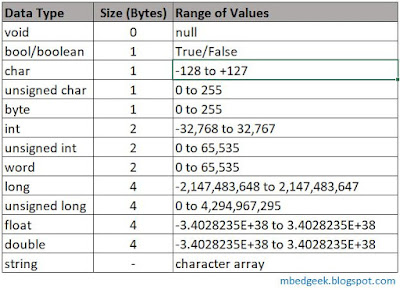Data Types in Arduino

### void

void is only used in Function Declaration. Functions with void return no values when they are called. However they can be used to do some tasks easily. Also it is important to note that Setup and Loop functions are of type void as they do not return any value.Setup and Loop functions do not return any value

### bool/boolean

'bool' is the standard data type defined in Arduino to hold a boolean value (either true or false). in some occasions people use this as 'boolean' also.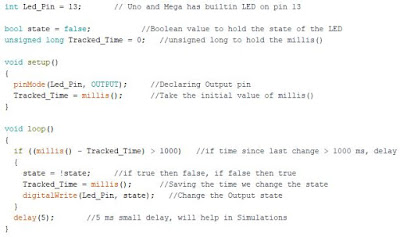Blink Code using boolean type variables
<Arduino Code>
```int Led_Pin = 13;       // Uno and Mega has builtin LED on pin 13

bool state = false;           //Boolean value to hold the state of the LED
unsigned long Tracked_Time = 0;   //unsigned long to hold the millis()

void setup()
{
pinMode(Led_Pin, OUTPUT);     //Declaring Output pin
Tracked_Time = millis();      //Take the initial value of millis()
}

void loop()
{
if ((millis() - Tracked_Time) > 1000)   //if time since last change > 1000 ms, delay
{
state = !state;     //if true then false, if false then true
Tracked_Time = millis();      //Saving the time we change the state
digitalWrite(Led_Pin, state);   //Change the Output state
}
delay(5);       //5 ms small delay, will help in Simulations
}
```

### char

Characters are stored in numbers. Therefore it is possible to do arithmetic operations on characters. The value used is the ASCII value of the Characters.char variable in Arduino

### unsigned char / byte

Unsigned char and byte in Arduino is all the same. These can be used to store values from 0 to 255.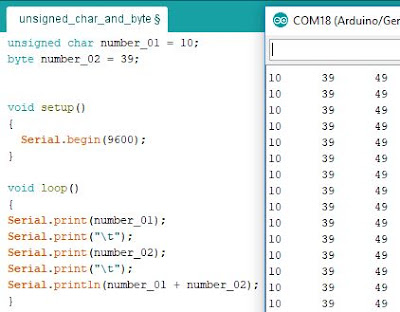unsigned char variable in Arduino

### int (integer)

Integer is the most used data type in Arduino. In ATmega based arduino board (Uno, Mega, Nano etc) 'int' uses 2 byte of memory and as a range of -32,768 to +32,767. But in some advanced boards like Due and MKR1000 int uses 4 bytes from memory and ranges from -2,147,483,648 to +2,147,483,647.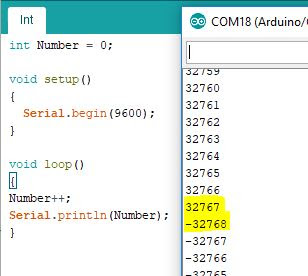int variable in Arduino

### unsigned int / word

unsigned int and word are very much same as int data type, instead they do not use the negative counter part of the variable. So the range of value becomes from 0 to 65,535. And same as Integer some boards like Arduino Due uses 4 byte of memory and the range becomes from 0 to 4,294,967,295.

### long and unsigned long

Long variables use 4 bytes from memory and unsigned long do not use the negative counterpart, so that positive range becomes larger. (refer Data Types Table for range of values)

It is very important to note use unsigned long variables when working with millis() function as millis() is an unsigned long variable. using other types may cause errors in arithmetic operations.

### float and double

In ATmega based boards float and double are all the same. However in some boards like Due double uses 8 byte of memory which gives precision of 64 bit. Mathematical operations with float values are not very efficient in Arduino. Therefore we may have to convert them to integers and do the operations when timing is very critical.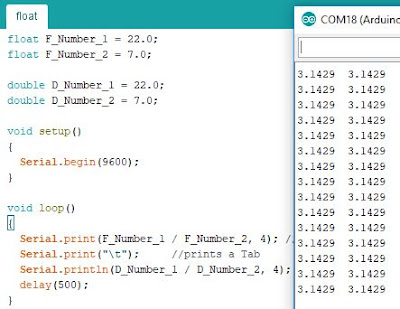float and double in Arduino

<Arduino Code>

```float F_Number_1 = 22.0;
float F_Number_2 = 7.0;

double D_Number_1 = 22.0;
double D_Number_2 = 7.0;

void setup()
{
Serial.begin(9600);
}

void loop()
{
Serial.print(F_Number_1 / F_Number_2, 4); //prints up to four decimal points
Serial.print("\t");     //prints a Tab
Serial.println(D_Number_1 / D_Number_2, 4);
delay(500);
}```

Arduino's are much slower in Arithmetic Operations with float variables compared to integer variables. Therefore there are occasions that we may need to even convert the float into integer and do the operations. See the below video to see the speed comparison in arithmetic operations and also serial print.

<Arduino Code>

```float num_1 = 21.0;   // Declare Variables
float num_2 = 7.0;
float num_Div = 0.0;

unsigned long Time_Start = 0;
unsigned long Time_Calculated = 0;

void setup()
{
Serial.begin(9600);
}

void loop()
{
Time_Start = millis();    //Record Starting Time
for (int i = 0; i < 1000; i++)    //Do a Small Calculation for 1000 iterations
{
num_1 = num_1 + 1.0;
num_Div = num_1 / num_2;
Serial.println(num_Div); //Serial Print the value of Calculation, use "Serial.println(num_Div,4);" to print with four decimal points
}
Time_Calculated = (millis() - Time_Start);
Serial.print("Calculated time for Float : ");
Serial.print(Time_Calculated);      //Print the Calculated time for 1000 iterations
Serial.println(" mS");

while(1)      //Stop Serial Print
{

}
}
```

### string

Strings in Arduino is a long subject to discuss and we'll be discussing it in a separate blog post.